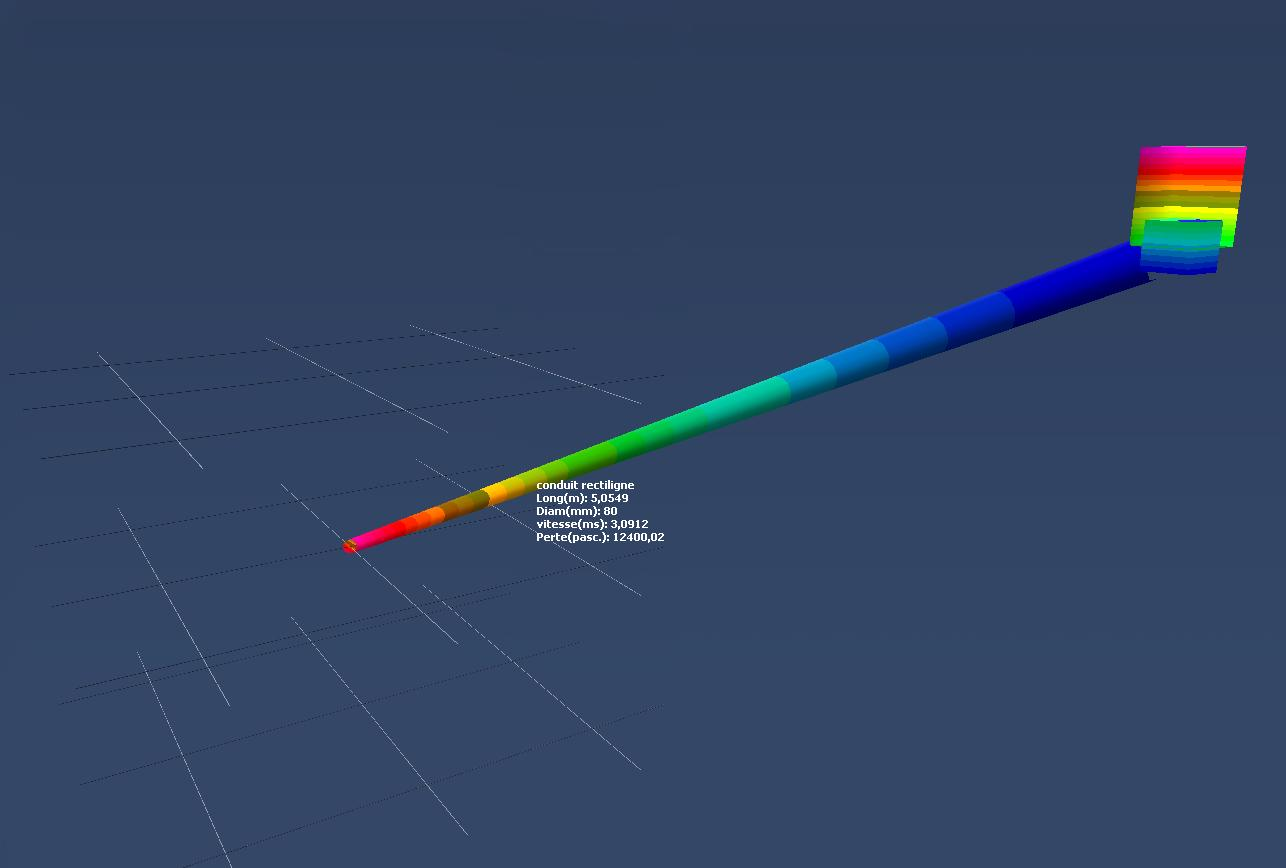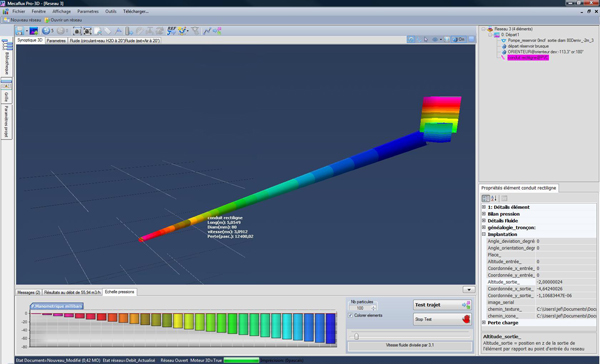• Calculate the flow in a pipe with a vertical drop (gravity flow):Here we explain the method of calculation of flow in a pipe with a slope (pressure losses are calculated with software or tables)

If the entry point of the network is higher than the outlet point, gravity flow is possible. This type of water intake generates a flow, depending on the three forms of energy pressure:

The flow in the conduit is reached, When the energy pressures are balanced between the entry point (A) and output (B) of the fluid (see Bernoulli):

with :

P.stat A= Static pressure at the entry point (pascals)

P.dyn A= Dynamic pressure at the entry point (pascals)

P.stat B= Static pressure at the outlet (pascals)

P.dyn B= Dynamic pressure at the outlet (pascals)

Pressure losses= Minor and Major head loss (pascals)

Energy in = energy out + energy lost

• Example of calculation of flow duct :
A water intake is connected to a pipe with an outlet point 2 meters below the point of entry. The diameter of the pipe is 80 mm ,its length is 5 meters and pipe roughness is 1mm. What is the flow rate of the installation?

The flow will be one that balances the equation:

P.stat A + p.dyn A = P.stat B + p.dyn B + head loss

Reminder:

dynamic pressure (pascal) = 0,5 x ρ x V²

Static pressure (pascal) = ρ x 9,81 x H

With :

ρ= Fluid density in Kg/M³ (for water will be taken ρ=1000 KG/m³)

V=fluid velocity m/sec = flow M³/h * section m²

H altitude with the zero point inlet (from the surface of the water intake)

At the input, the hydrostatic pressure is zero (Altitude = 0) and the dynamic pressure is zero (fluid velocity collected in a lake for example = 0)

therefore :

P.stat B + p.dyn B + head loss= 0

P.stat B= ρ x 9,81 x H = 1000 x 9,81 x (-2)= -19 620 pascals

it gives:

p.dynB + head loss= 19 620 pascals

The flow rate will be that which generates the pressure losses and a dynamic pressure being equal to the static pressure -19 620 pascals

p.dyn B = 0,5 x ρ x V² = 0,5 x 1000 x 9,81 x V²

head loss = Minor and Major head loss to the desired flow (we use tables or software to determine the major head loss in 5 meters pipe of roughness 1mm, and minor head loss will be neglected if we do not have software)

Some ittérations, calculations of losses, and dynamic pressures, taking the values of flow, allow us to determine the flow balancing equation: p.dynB + head loss= -19 620 pascals

Tutorial calculation flow network Mecaflux Pro 3D

The difficulty with this type of problem is :

• evaluation of head loss as it evolves according to the flow in a non-linear equation (see colebrook) which is solved with the Newton method or reading charts
• The necessity to repeatedly calculate to find the solution convergence, or draws a curve for pressure drop...

but here we will use a computer with mecaflux pro3D who perform these tasks in a few milliseconds: